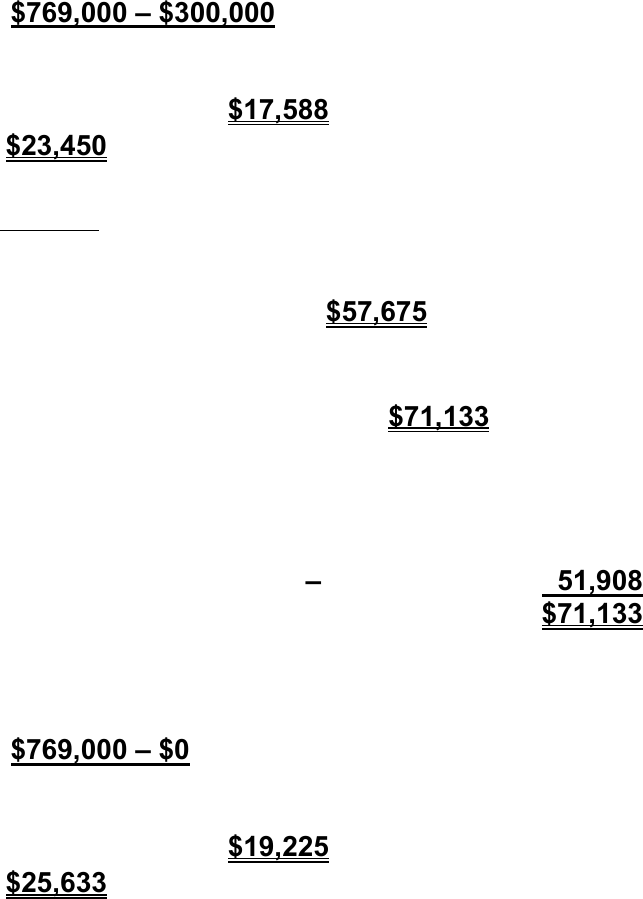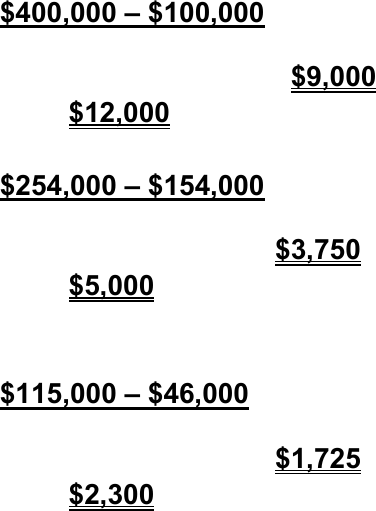Study Guides (390,000)
CA (150,000)
McMaster (9,000)
COMMERCE (1,000)
Min Hao (10)
Final

# COMMERCE 3AB3 Lecture Notes - Lecture 2: Book Value, Association To Advance Collegiate Schools Of Business, Input DeviceExam

Department
Commerce
Course Code
COMMERCE 3AB3
Professor
Min Hao
Study Guide
Final

This preview shows pages 1-3. to view the full 33 pages of the document.
EXERCISE 11.2
a. Total cost of property = \$220,000 + \$3,000 + \$1,500
= \$224,500
Cost of the building = \$224,500 X 75% = \$168,375
b. Depreciable amount = Cost Residual Value
= \$168,375 \$115,000 = \$53,375
c. Useful life is limited to 10 years. This is the number of
years the building will contribute economic benefits to the
company.
d. Depreciation expense 2020 (straight-line)
= (\$168,375 \$115,000) / 10 X 3.5 months/12 = \$1,557
e. Depreciation expense 2020 (double-declining)
= \$168,375 X 20%1 X 3.5 months/12 = \$9,822
Depreciation expense 2021 = (\$168,375 \$9,822) X 20%1
= \$31,711
1 Rate = (1 ÷ 10) X 2 = 20%
f. Carrying amount = \$168,375 \$9,822 \$31,711 = \$126,842
g. Under ASPE, depreciation expense is the higher of two amounts:
(1) cost less salvage value over the life of the asset, and (2) cost
less residual value over the asset’s useful life.
Under ASPE, depreciation expense 2020 (straight-line)
= (\$168,375 - \$0) / 20 X 3.5 months/12 = \$2,455
LO 2,3 BT: AP Difficulty: S Time: 20 min. AACSB: None CPA: cpa-t001 CM: Reporting

Only pages 1-3 are available for preview. Some parts have been intentionally blurred.EXERCISE 11.3
a.
\$769,000 \$300,000
= \$23,450 per year
20 years
2020: \$23,450 X 9/12 = \$17,588
2021: \$23,450
b.
100%
= 5%; 5% X 2 = 10%
20
9/12 X 10% X \$769,000 = \$57,675 for 2020
10% X (\$769,000 \$57,675) = \$71,133 for 2021
OR
3/12 X 10% X \$769,000
=
+
9/12 X 10% X (\$769,000 \$76,900)
=
for 2021
These two approaches will always yield the same result.
c.
\$769,000 \$0
= \$25,633 per year
30 years
2020: \$25,633 X 9/12 = \$19,225
2021: \$25,633
Under ASPE, depreciation expense is the higher of two amounts: (1)
cost less salvage value over the life of the asset, and (2) cost less
residual value over the asset’s useful life.
(1) (\$769,000 - \$0) / 30 = \$25,633
(2) (\$769,000 - \$300,000) / 20 = \$23,450

Only pages 1-3 are available for preview. Some parts have been intentionally blurred.EXERCISE 11.3 (CONTINUED)
a. It might be more appropriate to select the straight-line method if the
benefits of the asset are expected to flow to the entity evenly over
time, and if the decline in usefulness of the asset is expected to be
constant from period to period. It might be more appropriate to
select the double-declining-balance method if the greatest benefits
of the asset are expected to be yielded in the early years.
b. Under IFRS, depreciation expense is computed as follows:
Component 1:
\$400,000 \$100,000
= \$12,000 per year
25 years
2020: \$12,000 X 9/12 = \$9,000
2021: \$12,000
Component 2:
\$254,000 \$154,000
= \$5,000 per year
20 years
2020: \$5,000 X 9/12 = \$3,750
2021: \$5,000
Component 3:
\$115,000 \$46,000
= \$2,300 per year
30 years
2020: \$2,300 X 9/12 = \$1,725
2021: \$2,300
c. Under ASPE, the practice has been not to recognize asset
components to the same extent as under IFRS. Consequently, the
depreciation expense would be the same as calculated in c.
LO 3 BT: AP Difficulty: S Time: 35 min. AACSB: None CPA: cpa-t001 CM: Reporting